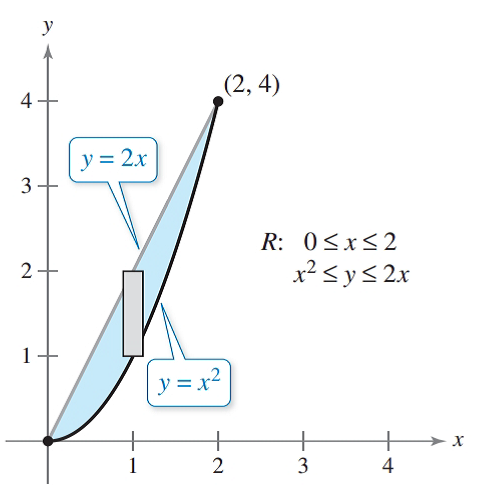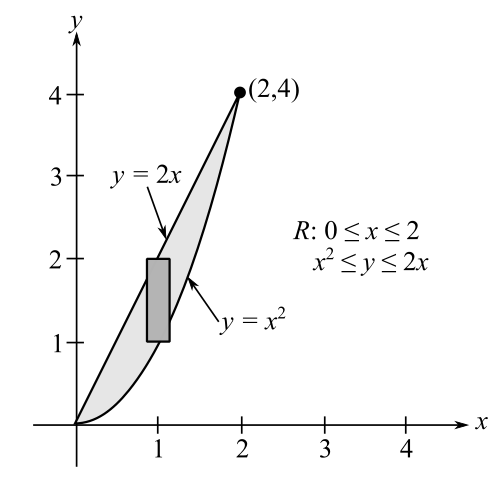Chapter 7.9, Problem 3CP### Calculus: An Applied Approach (Min...

10th Edition
Ron Larson
ISBN: 9781305860919

#### Solutions

Chapter
Section### Calculus: An Applied Approach (Min...

10th Edition
Ron Larson
ISBN: 9781305860919
Textbook Problem
1 views

# Find the volume of the solid region bounded above by the surface f ( x ,   y )   = 4 x 2 + 2 x y and below by the plane region R shown in the Figure.To determine

To calculate: The volume of the solid region bounded by the surface f(x,y)=4x2+2xy and the plane region as shown in the figure.Explanation

Given Information:

The provided function is f(x,y)=4x2+2xy.

And, the provided figure is,

Formula used:

Step 1: First decide the order of integration

If V=aby=f(x)x=g(x)f(x,y)dydx then take strip parallel to the y-axis.

If V=aby=f(x)x=g(y)f(x,y)dydx then take strip parallel to the x-axis.

Step 2: Integrate the function f(x,y).

If the order of integration dydx then integrate function first with respect to y and x constant

apply the limit. The integrate with respect x.

Step 3: Substitute the limit of x and get the volume of a given function.

Calculation:

The region R bounded by the parabola y=x2 and the line y=2x. So, the limits for y are x2y2x and the limits for x are 0x2.

The volume of the solid region is,

V=02x22xf(x,y)dydx=02x22x(4x2+2xy)dydx=02[4x2

### Still sussing out bartleby?

Check out a sample textbook solution.

See a sample solution

#### The Solution to Your Study Problems

Bartleby provides explanations to thousands of textbook problems written by our experts, many with advanced degrees!

Get Started

#### Find more solutions based on key concepts